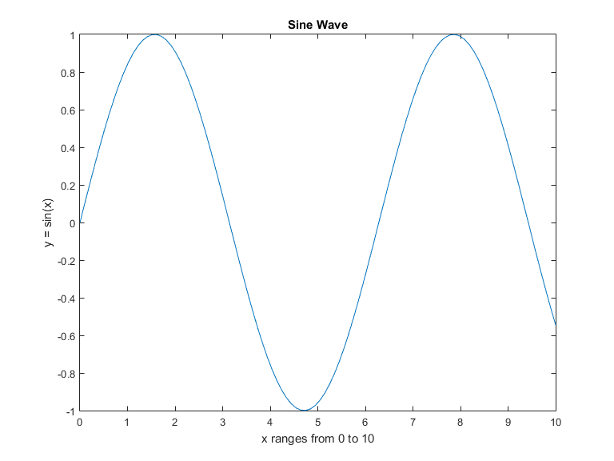## Save Figure to Reopen in MATLAB Later

This example shows how to save a figure so that you can reopen it in MATLAB® later. You can either save the figure to a FIG-file or you can generate and save the code.

### Save Figure to FIG-File

Create a plot to save. Add a title and axis labels.

```x = linspace(0,10); y = sin(x); plot(x,y) title('Sine Wave') xlabel('x ranges from 0 to 10') ylabel('y = sin(x)')```Save the figure to a FIG-file using the `savefig` function. The FIG-file stores the information required to recreate the figure.

```savefig('SineWave.fig') ```

Close the figure, then reopen the saved figure using the `openfig` function.

```close(gcf) openfig('SineWave.fig')```

`openfig` creates a new figure, a new axes, and a new line object using the same data as the original objects. Most of the property values of the new objects are the same as the original objects. However, any current default values apply to the new figure. You can interact with the figure. For example, you can pan, zoom, and rotate the axes.

Note

FIG-files open in MATLAB only. If you want to save the figure in a format that can be opened in another application, see Save Plot as Image or Vector Graphics File.

### Generate Code to Recreate Figure

Alternatively, generate the MATLAB code for the plot and then use the code to reproduce the graph. Generating the code captures modifications that you make using the plot tools.

Click File > Generate Code.... The generated code displays in the MATLAB Editor. Save the code by clicking File > Save As.

Generated files do not store the data necessary to recreate the graph, so you must supply the data arguments. The data arguments do not need to be identical to the original data. Comments at the beginning of the file state the type of data expected.